# Popularity hide and seek¶

"Estimate latent presidential popularity across time with a Markov chain"

• toc: true
• author: Alexandre Andorra, Rémi Louf
• categories: [popularity, Macron, Hidden Markov models, polls]
• image: images/hmm-popularity.png

A few months ago, I experimented with a Gaussian Process to estimate the popularity of French presidents across time. The experiment was really positive, and helped me get familiar with the beauty of GPs. This time, I teamed up with Rémi Louf on a Markov Chain model to estimate the same process -- what is the true latent popularity, that we only observe through the noisy data that are polls?

This was supposed to be a trial run before working on an electoral model for the coming regional elections in France -- it's always easier to start with 2 dimensions than 6, right? But the model turned out to be so good at smoothing and predicting popularity data that we thought it'd be a shame not to share it. And voilà!

## Show me the data!¶

The data are the same as in my GP post, so we're not going to spend a lot of time explaining them. It's basically all the popularity opinion polls of French presidents since the term limits switched to 5 years (in 2002).

Let's import those data, as well as the (fabulous) packages we'll need:

In :
import datetime

import arviz
import matplotlib.pyplot as plt
import numpy as np
import pandas as pd
import pymc3 as pm
import theano.tensor as aet
from scipy.special import expit as logistic

In :
# hide
RANDOM_SEED = 926
np.random.seed(RANDOM_SEED)
arviz.style.use("arviz-darkgrid")

In :
data = pd.read_csv(
"https://raw.githubusercontent.com/AlexAndorra/pollsposition_models/master/data/raw_popularity_presidents.csv",
index_col=0,
parse_dates=True,
)

In :
# hide
# restrict data to after the switch to 5-year term
data = data.loc[data.index >= pd.to_datetime("2002-05-05")]
data["year"] = data.index.year
data["month"] = data.index.month

data["sondage"] = data["sondage"].replace("Yougov", "YouGov")
data["method"] = data["method"].replace("face-to-face&internet", "face to face")

# convert to proportions
data[["approve_pr", "disapprove_pr"]] = (
data[["approve_pr", "disapprove_pr"]].copy() / 100
)
data = data.rename(columns={"approve_pr": "p_approve", "disapprove_pr": "p_disapprove"})

POLLSTERS = data["sondage"].sort_values().unique()
comment = f"""The dataset contains {len(data)} polls between the years {data["year"].min()} and {data["year"].max()}.
There are {len(POLLSTERS)} pollsters: {', '.join(list(POLLSTERS))}
"""
print(comment)

The dataset contains 1083 polls between the years 2002 and 2021.
There are 10 pollsters: BVA, Elabe, Harris, Ifop, Ipsos, Kantar, Odoxa, OpinionWay, Viavoice, YouGov



The number of polls is homogeneous among months, except in the summer because, well, France:

In :
data["month"].value_counts().sort_index()

Out:
1     100
2      96
3     100
4      89
5      91
6      95
7      68
8      71
9      94
10     99
11     98
12     82
Name: month, dtype: int64

Let us look at simple stats on the pollsters:

In :
pd.crosstab(data.sondage, data.method, margins=True)

Out:
method face to face internet phone phone&internet All
sondage
BVA 0 101 89 0 190
Elabe 0 52 0 0 52
Harris 0 33 0 0 33
Ifop 0 29 181 38 248
Ipsos 0 40 177 0 217
Kantar 208 4 0 0 212
Odoxa 0 67 0 0 67
OpinionWay 0 12 0 0 12
Viavoice 0 20 0 0 20
YouGov 0 32 0 0 32
All 208 390 447 38 1083

Interesting: most pollsters only use one method -- internet. Only BVA, Ifop, Ipsos (and Kantar very recently) use different methods. So, if we naively estimate the biases of pollsters and methods individually, we'll get high correlations in our posterior estimates -- the parameter for face to face will basically be the one for Kantar, and vice versa. So we will need to model the pairs (pollster, method) rather than pollsters and methods individually.

Now, let's just plot the raw data and see what they look like:

In :
approval_rates = data["p_approve"].values
disapproval_rates = data["p_disapprove"].values
doesnotrespond = 1 - approval_rates - disapproval_rates
newterm_dates = data.reset_index().groupby("president").first()["index"].values
dates = data.index

fig, axes = plt.subplots(2, figsize=(12, 6))
for ax, rate, label in zip(
axes.ravel(),
[approval_rates, doesnotrespond],
):
ax.plot(dates, rate, "o", alpha=0.4)
ax.set_ylim(0, 1)
ax.set_ylabel(label)
for date in newterm_dates:
ax.axvline(date, color="k", alpha=0.6, linestyle="--")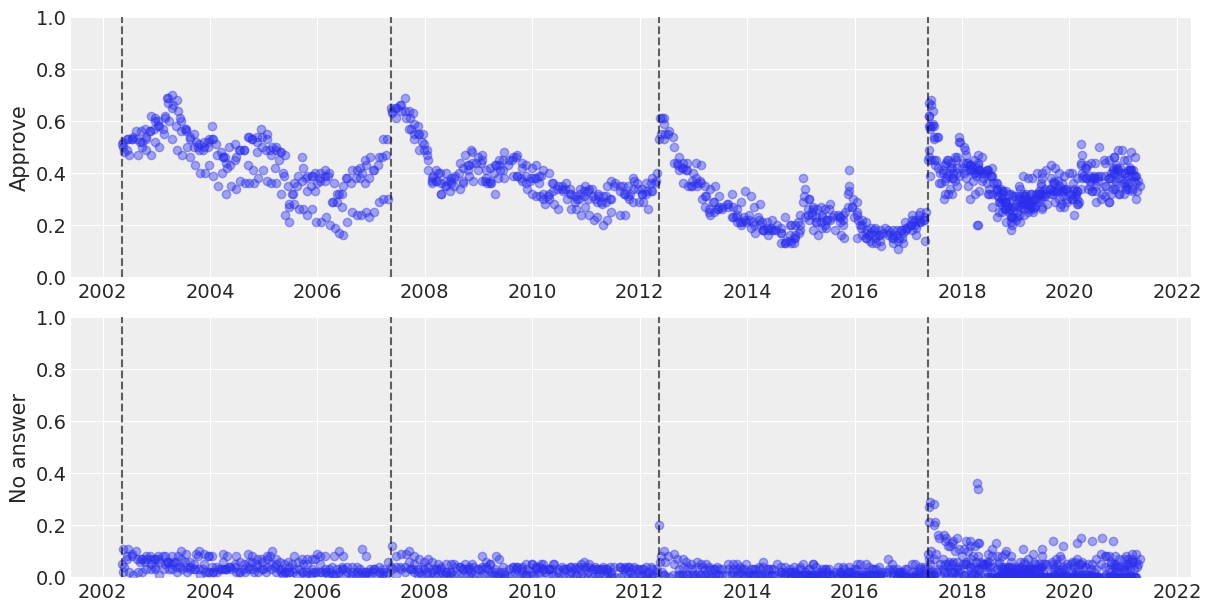We notice two things when looking at these plots:

1. Approval rates systematically decrease as the goes on.
2. While that's true, some events seem to push the approval rate back up, even though temporarily. This happened in every term, actually. Can that variance really be explained solely with a random walk?
3. Non-response rate is higher during Macron's term.

## Monthly standard deviation¶

Something that often proves challenging with count data is that they are often more dispersed than traditional models expect them to be. Let's check this now, by computing the monthly standard deviation of the approval rates (we weigh each poll equally, even though we probably should weigh them according to their respective sample size):

In :
rolling_std = (
data.reset_index()
.groupby(["year", "month"])
.std()
.reset_index()[["year", "month", "p_approve"]]
)
rolling_std

Out:
year month p_approve
0 2002 5 0.017078
1 2002 6 0.030000
2 2002 7 0.005774
3 2002 8 0.045826
4 2002 9 0.025166
... ... ... ...
223 2020 12 0.064627
224 2021 1 0.042661
225 2021 2 0.041748
226 2021 3 0.042980
227 2021 4 0.020000

228 rows × 3 columns

In :
fig, ax = plt.subplots(figsize=(10, 4))
ax.plot(
pd.to_datetime(
[f"{y}-{m}-01" for y, m in zip(rolling_std.year, rolling_std.month)]
),
rolling_std.p_approve.values,
"o",
alpha=0.5,
)
ax.set_title("Monthly standard deviation in polls")
for date in newterm_dates:
ax.axvline(date, color="k", alpha=0.6, linestyle="--")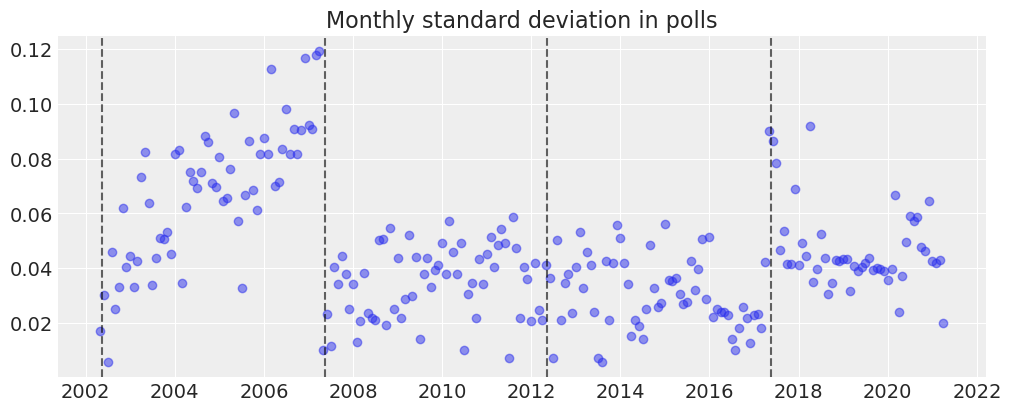There is a very high variance for Chirac's second term, and for the beggining of Macron's term. For Chirac's term, it seems like the difference stems from the polling method: face-to-face approval rates seem to be much lower, as you can see in the figure below. For Macron, this high variance is quite hard to explain. In any case, we'll probably have to take this overdispersion (as it's called in statistical linguo) of the data in our models...

In :
face = data[data["method"] == "face to face"]
dates_face = face.index

other = data[data["method"] != "face to face"]
dates_other = other.index

fig, ax = plt.subplots(figsize=(10, 4))
ax.plot(dates_face, face["p_approve"].values, "o", alpha=0.3, label="face to face")
ax.plot(dates_other, other["p_approve"].values, "o", alpha=0.3, label="other")
ax.set_ylim(0, 1)
ax.set_ylabel("Does approve")
ax.set_title("Raw approval polls")
ax.legend()
for date in newterm_dates:
ax.axvline(date, color="k", alpha=0.6, linestyle="--")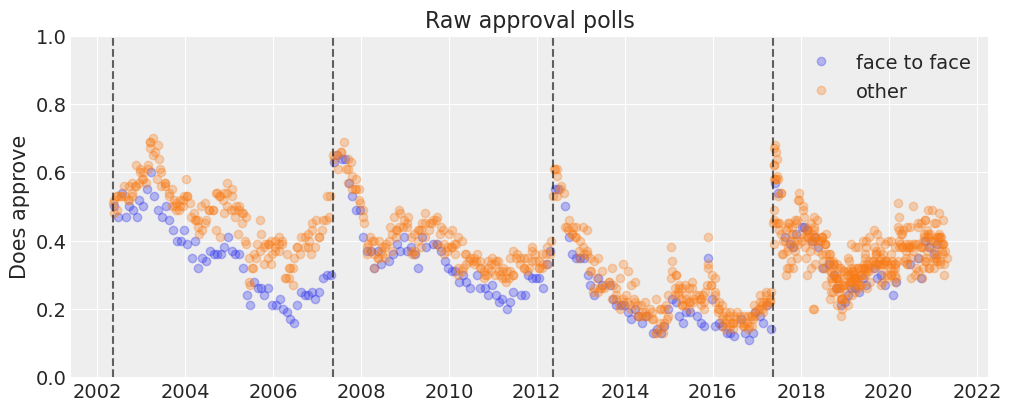## A raw analysis of bias¶

As each pollster uses different methods to establish and question their samples each month, we don't expect their results to be identical -- that would be troubling. Instead we expect each pollster and each polling method to be at a different place on the spectrum: some report popularity rates in line with the market average, some are below average, some are above.

The model will be able to estimate this bias on the fly and more seriously (if we tell it to), but let's take a look at a crude estimation ourselves, to get a first idea. Note that we're talking about statistical bias here, not political bias: it's very probable that reaching out to people only by internet or phone can have a selection effect on your sample, without it being politically motivated -- statistics are just hard and stubborn you know 🤷‍♂️

To investigate bias, we now compute the monthly mean of the $p_{approve}$ values and check how each individual poll strayed from this mean:

In :
data = (
data.reset_index()
.merge(
data.groupby(["year", "month"])["p_approve"].mean().reset_index(),
on=["year", "month"],
suffixes=["", "_mean"],
)
.rename(columns={"index": "field_date"})
)
data["diff_approval"] = data["p_approve"] - data["p_approve_mean"]
data.round(2)

Out:
field_date president sondage samplesize method p_approve p_disapprove year month p_approve_mean diff_approval
0 2002-05-15 chirac2 Ifop 924 phone 0.51 0.44 2002 5 0.50 0.01
1 2002-05-20 chirac2 Kantar 972 face to face 0.50 0.48 2002 5 0.50 -0.00
2 2002-05-23 chirac2 BVA 1054 phone 0.52 0.37 2002 5 0.50 0.02
3 2002-05-26 chirac2 Ipsos 907 phone 0.48 0.48 2002 5 0.50 -0.02
4 2002-06-16 chirac2 Ifop 974 phone 0.49 0.43 2002 6 0.50 -0.02
... ... ... ... ... ... ... ... ... ... ... ...
1078 2021-03-29 macron Kantar 1000 internet 0.36 0.58 2021 3 0.38 -0.02
1079 2021-03-30 macron YouGov 1068 internet 0.30 0.61 2021 3 0.38 -0.08
1080 2021-04-07 macron Elabe 1003 internet 0.33 0.63 2021 4 0.35 -0.02
1081 2021-04-10 macron Ipsos 1002 internet 0.37 0.58 2021 4 0.35 0.02
1082 2021-04-26 macron Kantar 1000 internet 0.35 0.58 2021 4 0.35 0.00

1083 rows × 11 columns

Then, we can aggregate these offsets by pollster and look at their distributions:

In :
POLLSTER_VALS = {
pollster: data[data["sondage"] == pollster]["diff_approval"].values
for pollster in list(POLLSTERS)
}

colors = plt.rcParams["axes.prop_cycle"]()
fig, axes = plt.subplots(ncols=2, nrows=5, sharex=True, figsize=(12, 12))

for ax, (pollster, vals) in zip(axes.ravel(), POLLSTER_VALS.items()):
c = next(colors)["color"]
ax.hist(vals, alpha=0.3, color=c, label=pollster)
ax.axvline(x=np.mean(vals), color=c, linestyle="--")
ax.axvline(x=0, color="black")
ax.set_xlim(-0.3, 0.3)
ax.legend()

plt.xlabel(r"$p_{approve} - \bar{p}_{approve}$", fontsize=25);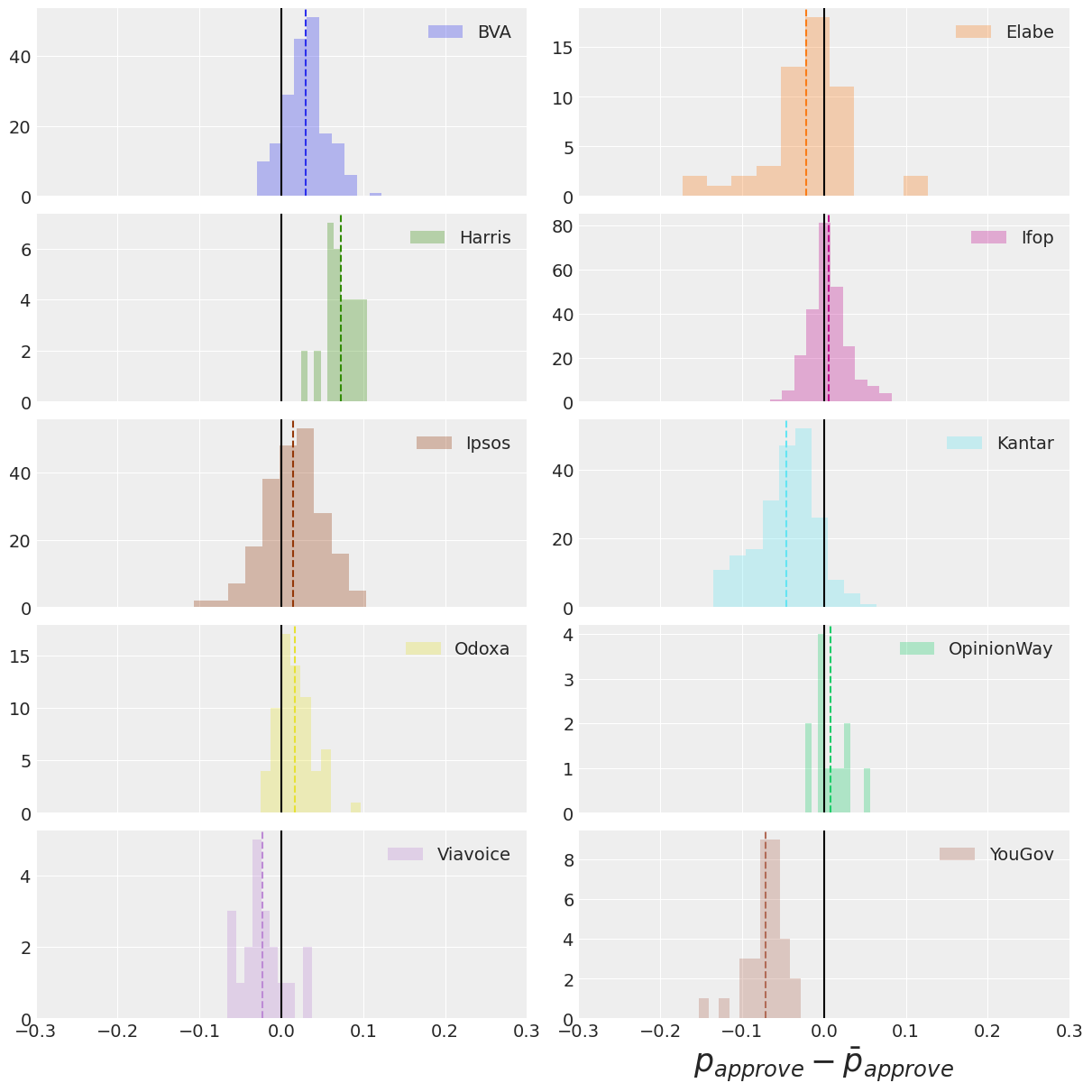A positive (resp. negative) bias means the pollster tends to report higher (resp. lower) popularity rates than the average pollster. We'll see what the model has to say about this, but our prior is that, for instance, YouGov and Kantar tend to be below average, while Harris and BVA tend to be higher.

And now for the bias per method:

In :
METHOD_VALS = {
method: data[data["method"] == method]["diff_approval"].values
for method in list(data["method"].unique())
}

colors = plt.rcParams["axes.prop_cycle"]()
fig, ax = plt.subplots(figsize=(11, 5))

for method, vals in METHOD_VALS.items():
c = next(colors)["color"]
ax.hist(vals, alpha=0.3, color=c, label=method)
ax.axvline(x=np.mean(vals), color=c, linestyle="--")

ax.axvline(x=0, color="black")
ax.set_xlim(-0.2, 0.2)
ax.set_xlabel(r"$p_+ - \bar{p}_{+}$", fontsize=25)
ax.legend();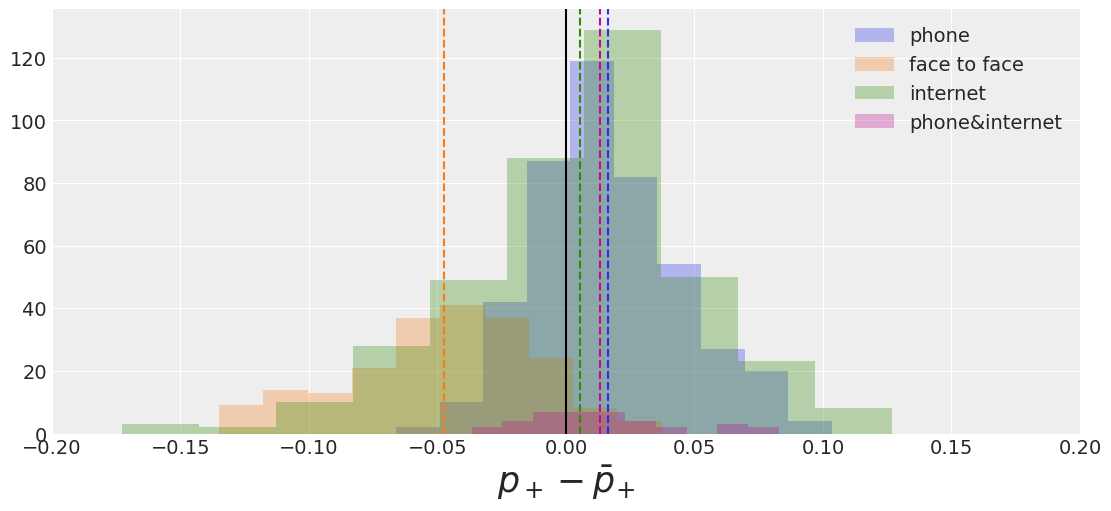Face-to-face polls seem to give systematically below-average approval rates, while telephone polls seem to give slightly higher-than-average results.

Again, keep in mind that there is substantial correlation between pollsters and method, so take this with a grain of salt -- that's why it's useful to add that to the model actually: it will be able to decipher these correlations, integrate them into the full data generating process, and report finer estimates of each bias.

Speaking of models, do you know what time it is? It's model time, of course!!

## Specifying the model¶

We'll build several versions of our model, refining it incrementally. But the basic structure will remain the same. Let's build an abstract version that will help you understand the code.

Each poll $i$ at month $m$ from the beginning of a president’s term finds that $y_i$ individuals have a positive opinion of the president’s action over $n_i$ respondents. We model this as:

$$y_{i,m} \sim Binomial(p_{i,m}, n_{i,m})$$

We loosely call $p_{i,m}$ the popularity of the president in poll $i$, $m$ months into his presidency.

Why specify the month when the time information is already contained in the succession of polls? Because French people tend to be less and less satisfied with their president as their term moves, regardless of their action -- you'll see...

We model $p_{i,m}$ with a random walk logistic regression:

$$p_{i,m} = logistic(\mu_m + \alpha_k + \zeta_j)$$

$\mu_m$ is the latent support for the president at month $m$ and it's the main quantity we would like to model. $\alpha_k$ is the bias of the pollster, while $\zeta_j$ is the inherent bias of the polling method. The biases are assumed to be completely unpooled at first, i.e we model one bias for each pollster and method:

$$\alpha_k \sim Normal(0, \sigma_k)\qquad \forall \, pollster \, k$$

and

$$\zeta_j \sim Normal(0, \sigma_j)\qquad \forall \, method \, j$$

We treat the time variation of $\mu$ with a correlated random walk:

$$\mu_m | \mu_{m-1} \sim Normal(\mu_{m-1}, \sigma_m)$$

Again, note that $\mu$ is latent: we never get to observe it in the world.

For the sake of simplicity, we choose not to account at first for a natural decline in popularity $\delta$, the unmeployment at month $m$, or random events that can happen during the term.

### Mark What ?¶

Thus defined, our model is a Markov Model. This is a big and scary word to describe what is actually a simple concept (tip: this is a common technique to make us statisticians look cool and knowledgeable): a model where a "hidden" state jumps from one time step to another and where the observations are a function of this hidden state. Hidden states have no memory, in the sense that their value at any time step only depends on the value of the state at the previous time step. That's what markovian means.

Here, the hidden state is the latent popularity $\mu_m$ and we combine it with the effects $\alpha_k$ and $\zeta_j$ to compute the value of the observed states, the polling results $y_{i,m}$. The value of the latent popularity at month $m$ only depends on its value at $m-1$, and the jumps between months are normally distributed.

In :
# hide
data["num_approve"] = np.floor(data["samplesize"] * data["p_approve"]).astype("int")
data

Out:
field_date president sondage samplesize method p_approve p_disapprove year month p_approve_mean diff_approval num_approve
0 2002-05-15 chirac2 Ifop 924 phone 0.51 0.44 2002 5 0.502500 7.500000e-03 471
1 2002-05-20 chirac2 Kantar 972 face to face 0.50 0.48 2002 5 0.502500 -2.500000e-03 486
2 2002-05-23 chirac2 BVA 1054 phone 0.52 0.37 2002 5 0.502500 1.750000e-02 548
3 2002-05-26 chirac2 Ipsos 907 phone 0.48 0.48 2002 5 0.502500 -2.250000e-02 435
4 2002-06-16 chirac2 Ifop 974 phone 0.49 0.43 2002 6 0.505000 -1.500000e-02 477
... ... ... ... ... ... ... ... ... ... ... ... ...
1078 2021-03-29 macron Kantar 1000 internet 0.36 0.58 2021 3 0.375455 -1.545455e-02 360
1079 2021-03-30 macron YouGov 1068 internet 0.30 0.61 2021 3 0.375455 -7.545455e-02 320
1080 2021-04-07 macron Elabe 1003 internet 0.33 0.63 2021 4 0.350000 -2.000000e-02 330
1081 2021-04-10 macron Ipsos 1002 internet 0.37 0.58 2021 4 0.350000 2.000000e-02 370
1082 2021-04-26 macron Kantar 1000 internet 0.35 0.58 2021 4 0.350000 5.551115e-17 350

1083 rows × 12 columns

To define our model, we'll use PyMC's named coordinates feature. That way, we'll be able to write down our model using the names of variables instead of their shape dimensions. To do that, we need to define a bunch of variables:

In :
pollster_by_method_id, pollster_by_methods = data.set_index(
["sondage", "method"]
).index.factorize(sort=True)
month_id = np.hstack(
[
pd.Categorical(
data[data.president == president].field_date.dt.to_period("M")
).codes
for president in data.president.unique()
]
)
months = np.arange(max(month_id) + 1)
data["month_id"] = month_id

In :
COORDS = {
"pollster_by_method": pollster_by_methods,
"month": months,
# each observation is uniquely identified by (pollster, field_date):
"observation": data.set_index(["sondage", "field_date"]).index,
}


### Fixed sigma for the random walk¶

Our first model is as simple as possible: just a random walk on the monthly latent popularity and a term for the bias of each (pollster, method) pair, which is called the "house effect" in the political science litterature. Also, we'll use a more descriptive name for $\mu$ -- month_effect sounds good, because, well, that's basically what it is. We'll arbitrarily fix the innovation of the random walk (sigma) to 1 and see how it fares.

In :
with pm.Model(coords=COORDS) as pooled_popularity_simple:

house_effect = pm.Normal("house_effect", 0, 0.15, dims="pollster_by_method")
month_effect = pm.GaussianRandomWalk("month_effect", sigma=1.0, dims="month")

popularity = pm.math.invlogit(
month_effect[month_id] + house_effect[pollster_by_method_id]
)

N_approve = pm.Binomial(
"N_approve",
p=popularity,
n=data["samplesize"],
observed=data["num_approve"],
dims="observation",
)

idata = pm.sample(return_inferencedata=True)

Auto-assigning NUTS sampler...
Initializing NUTS using jitter+adapt_diag...
Multiprocess sampling (4 chains in 4 jobs)
NUTS: [month_effect, house_effect]

100.00% [8000/8000 00:23<00:00 Sampling 4 chains, 0 divergences]
Sampling 4 chains for 1_000 tune and 1_000 draw iterations (4_000 + 4_000 draws total) took 42 seconds.

0, dim: observation, 1083 =? 1083

The acceptance probability does not match the target. It is 0.3442984443078715, but should be close to 0.8. Try to increase the number of tuning steps.
The rhat statistic is larger than 1.05 for some parameters. This indicates slight problems during sampling.
The estimated number of effective samples is smaller than 200 for some parameters.


We plot the posterior distribution of the pollster and method biases:

In :
arviz.plot_trace(idata);